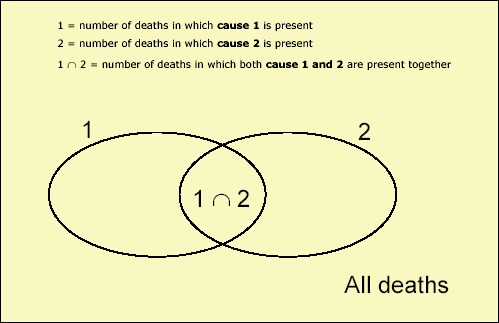3319.0.55.001 - Multiple Cause of Death Analysis, 1997-2001
ARCHIVED ISSUE Released at 11:30 AM (CANBERRA TIME) 22/07/2003
 Page tools: .mffeedback,#pjs { display :none; } Enable Javascript to Print PagesPrint AllEmail Notification Further Examples of Ratio of Observed to Expected Joint Frequencies Tables (Appendix) The Death Certificate (Appendix) Multiple Cause of Death Analysis Currently Undertaken by ABS (Appendix) Method of Calculating Observed to Expected Ratio as a Measure of Strength of Association (Appendix) List of References (Appendix) APPENDIX V. METHOD OF CALCULATING OBSERVED TO EXPECTED RATIO AS A MEASURE OF STRENGTH OF ASSOCIATION Note that this technique uses counts of causes by deaths, not by mentions (see Glossary, Appendix III). The totals needed to compute this ratio are as follows (each number relates to the specific time period and the sex and age group being examined): i) actual number of deaths with the pair of causes (cause 1 & cause 2) ii) total number of deaths where cause 1 is present iii) total number of deaths where cause 2 is present iv) total number of deaths from all causes For all of these numbers, multiple cause data are being used. Thus, for i) above we are counting the number of deaths in which both causes appear somewhere on the death certificate - neither is necessarily the underlying cause.The expected frequency can be ascertained by multiplying the number of deaths in which cause 1 is present by the number of deaths in which cause 2 is present, and then dividing this product by the total number of deaths in that time period and demographic. This number represents the frequency with which the two causes would be expected to appear together if there was no association between the causes. A ratio of observed to expected joint frequency can then be calculated. Worked example (diabetes mellitus(E10-E14) and Ischaemic heart diseases(I20-I25)): This example is using data from 1997-2001 (for all ages) coded to ICD-10 Actual number of deaths with both Ischaemic heart diseases and diabetes = 24543 Total number of deaths with diabetes present = 49,012 Total number of deaths with Ischaemic heart diseases present = 190,524 Total number of deaths from all causes = 641,489 Expected number of deaths with both causes (assuming independence) = (49,012 x 190,524) / 641,489 = 14556.7 Ratio actual/expected = 24543/14556.7 = 1.69 Thus, diabetes appears on death certificates in conjunction with ischaemic heart diseases more frequently (1.69 times more often) than would be expected if the two diseases were independent. Back To Publication Document Selection These documents will be presented in a new window.